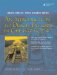The signature of a function consists of its name and its parameter list. In C++, the return type is not part of the signature.

C++ permits overloading of function names. A function name is overloaded if it has more than one meaning within a given scope. Overloading occurs when two or more functions within a given scope have the same name but different signatures. It is an error to have two functions in the same scope with the same signature but different return types.

Function Call Resolution

When a function call is made to an overloaded function within a given scope, the C++ compiler determines from the arguments which version of the function to invoke. To do this, a match must be found between the number and type of the arguments and the signature of exactly one of the overloaded functions. This is the sequence of steps that the compiler takes to determine which overloaded function to call.

 1 If there is an exact match with one function, call it. 2 Else, match through standard type promotions (see Section 19.5). 3 Else, match through conversion constructors or conversion operators (see Section 2.12). 4 Else, match through ellipsis (...) (see Section 24.1), if found 5 Else, report an error.

Example 5.1 shows a class with six member functions, each with a distinct signature. Keep in mind that each function has an additional implicit parameter: this. The keyword const, following the parameter list, protects the host object from the action of the function and is part of its signature.

Example 5.1. src/functions/function-call.cpp

 ```[ . . . . ] class SignatureDemo { public: SignatureDemo(int val) : m_Val(val) {} void demo(int n) {cout << ++m_Val << " demo(int)" << endl;} void demo(int n) const <-- 1 {cout << m_Val << " demo(int) const" << endl;} /* void demo(const int& n) {cout << ++m_Val << " demo(int&)" << endl;} */ <-- 2 void demo(short s) {cout << ++m_Val << " demo(short)" << endl;} void demo(float f) {cout << ++m_Val << " demo(float)" << endl;} void demo(float f) const {cout << m_Val << " demo(float) const" << endl;} void demo(double d) {cout << ++m_Val << " demo(double)" << endl;} private: int m_Val; }; ```   (1)overloaded on const-ness (2)clashes with previous function

Example 5.2 contains some client code that tests the overloaded functions from SignatureDemo.

Example 5.2. src/functions/function-call.cpp

 ```[ . . . . ] int main() { SignatureDemo sd(5); const SignatureDemo csd(17); sd.demo(2); csd.demo(2); <-- 1 int i = 3; sd.demo(i); short s = 5; sd.demo(s); csd.demo(s); <-- 2 sd.demo(2.3); <-- 3 float f(4.5); sd.demo(f); csd.demo(f); csd.demo(4.5); return 0; } ```   (1)const version is called. (2)Non-const short cannot be called, so a promotion to int is required to call the const int version. (3)This is double, not float.

The output should look something like this:

```6 demo(int)
17 demo(int) const
7 demo(int)
8 demo(short)
17 demo(int) const
9 demo(double)
10 demo(float)
17 demo(float) const
```

 1 Experiment with Example 5.1. Start by uncommenting the third member function and compiling. 2 Try adding the following line just before the end of main(): `csd.demo(4.5);`   What happened? Explain the error message. 3 Add other function calls and other variations on the demo() function. Explain each result.An Introduction to Design Patterns in C++ with Qt 4
ISBN: 0131879057
EAN: 2147483647
Year: 2004
Pages: 268